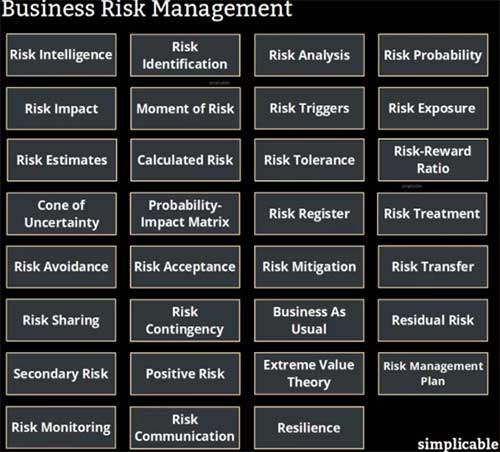CFA一级考试攻略：贝叶斯法则

cfa2019-05-13 17:57 浏览量: 150

CFA一级考试攻略：贝叶斯法则。复习到概率部分，就不得不提大名鼎鼎的贝叶斯法则啦~但是在学习贝叶斯法则前，我们还需要了解一些基础的概念。CFA考试比如说独立和互斥事件，还有全概率的计算。下面欣欣老师就带大家来一一掌握。

CFA一级考试的独立事件和互斥事件

1independent events 独立事件

（1）理解：A和B的发生是互不影响的，A、B发不发生，跟对方都没有关系。

（2）公式：P(B|A)=P(B) P(A|B)=P(A) P(AB)= P(A)P(B)

2mutually exclusive events 互斥事件

（1）理解：A和B不能同时发生，互斥一定不独立。

（2）公式：P(AB)=P(A|B) =P(B|A)=0 P(A or B)=P(A)+P(B)

Total probability rule

CFA全概率计算

1公式

For unconditional probability of event A,P(A)=P(A|S1 )P(S1 )+P(A|S2 )P(S2 )+……+P(A|S

CFA考试相关文章阅读推荐：

cfa考试二级考纲变动分析

2020年CFA考试零基础难不难，解答

CFA考试计算机规定两款

CFA持证人福利有哪些？北上广深

2019frm含金量怎么样

•快捷入口

• CFA证书• CFA考点• CFA费用